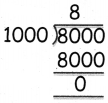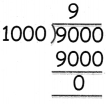Tamilnadu State Board New Syllabus Samacheer Kalvi 4th Maths Guide Pdf Term 3 Chapter 3 Measurements Ex 3.1 Textbook Questions and Answers, Notes.

## Tamilnadu Samacheer Kalvi 4th Maths Solutions Term 3 Chapter 3 Measurements Ex 3.1

Question 1.
Rani hod 1 litre coconut oil. She shared it equally among her 5 friends. How much does each person have?
1 litre = 1000 ml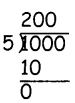Each person have 200 ml.Question 2.
A teapot contains 2 litres, it is poured in cups with a capacity of 500 ml. How many cups can be filled?
1 litre = 1000 ml
2 litre = 1000 × 2
= 2000 ml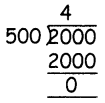4 cups

Question 3.
Ram has 1 litre of juice bottle if he gives his friend 100 ml of juice. How much is left with him?
1 litre = 1000 ml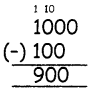900 ml is left with meQuestion 4.
Change litre into millilitre
1. 1l = ______ ml
1l = 1000 ml

2. 7l = ______ ml
7l = 7 × 1000 = 7000 ml

3. 5l = ______ ml
5l = 5 × 1000 = 5000 ml4. 9l = ______ ml
9l = 9 × 1000 = 9000 ml

5. 4l = ______ ml
4l = 4 × 1000 = 4000 ml

Question 5.
Change millilitre into litre
1. 6000 ml = ______ l
6 litres2. 2000 ml = ______ l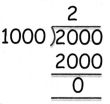2 litres

3. 8000 ml = ______l